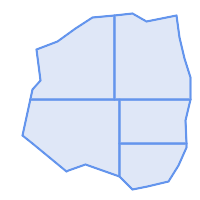## Name

ST_Subdivide — Computes a rectilinear subdivision of a geometry.

## Synopsis

`setof geometry ST_Subdivide(`geometry geom, integer max_vertices=256, float8 gridSize = -1`)`;

## Description

Returns a set of geometries that are the result of dividing `geom` into parts using rectilinear lines, with each part containing no more than `max_vertices`.

`max_vertices` must be 5 or more, as 5 points are needed to represent a closed box. `gridSize` can be specified to have clipping work in fixed-precision space (requires GEOS-3.9.0+).

Point-in-polygon and other spatial operations are normally faster for indexed subdivided datasets. Since the bounding boxes for the parts usually cover a smaller area than the original geometry bbox, index queries produce fewer "hit" cases. The "hit" cases are faster because the spatial operations executed by the index recheck process fewer points.This is a set-returning function (SRF) that return a set of rows containing single geometry values. It can be used in a SELECT list or a FROM clause to produce a result set with one record for each result geometry.

Performed by the GEOS module.

Availability: 2.2.0

Enhanced: 2.5.0 reuses existing points on polygon split, vertex count is lowered from 8 to 5.

Enhanced: 3.1.0 accept a gridSize parameter, requires GEOS >= 3.9.0 to use this new feature.

## Examples

Example: Subdivide a polygon into parts with no more than 10 vertices, and assign each part a unique id.Subdivided to maximum 10 vertices

```SELECT row_number() OVER() As rn, ST_AsText(geom) As wkt
FROM (SELECT ST_SubDivide(
'POLYGON((132 10,119 23,85 35,68 29,66 28,49 42,32 56,22 64,32 110,40 119,36 150,
57 158,75 171,92 182,114 184,132 186,146 178,176 184,179 162,184 141,190 122,
190 100,185 79,186 56,186 52,178 34,168 18,147 13,132 10))'::geometry,10))  AS f(geom);
```
``` rn │                                                      wkt
────┼────────────────────────────────────────────────────────────────────────────────────────────────────────────────
1 │ POLYGON((119 23,85 35,68 29,66 28,32 56,22 64,29.8260869565217 100,119 100,119 23))
2 │ POLYGON((132 10,119 23,119 56,186 56,186 52,178 34,168 18,147 13,132 10))
3 │ POLYGON((119 56,119 100,190 100,185 79,186 56,119 56))
4 │ POLYGON((29.8260869565217 100,32 110,40 119,36 150,57 158,75 171,92 182,114 184,114 100,29.8260869565217 100))
5 │ POLYGON((114 184,132 186,146 178,176 184,179 162,184 141,190 122,190 100,114 100,114 184))
```

Example: Densify a long geography line using ST_Segmentize(geography, distance), and use ST_Subdivide to split the resulting line into sublines of 8 vertices.The densified and split lines.

```SELECT ST_AsText( ST_Subdivide(
ST_Segmentize('LINESTRING(0 0, 85 85)'::geography,
1200000)::geometry,    8));
```
```LINESTRING(0 0,0.487578359029357 5.57659056746196,0.984542144675897 11.1527721155093,1.50101059639722 16.7281035483571,1.94532113630331 21.25)
LINESTRING(1.94532113630331 21.25,2.04869538062779 22.3020741387339,2.64204641967673 27.8740533545155,3.29994062412787 33.443216802941,4.04836719489742 39.0084282520239,4.59890468420694 42.5)
LINESTRING(4.59890468420694 42.5,4.92498503922732 44.5680389206321,5.98737409390639 50.1195229244701,7.3290919767674 55.6587646879025,8.79638749938413 60.1969505994924)
LINESTRING(8.79638749938413 60.1969505994924,9.11375579533779 61.1785363177625,11.6558166691368 66.6648504160202,15.642041247655 72.0867690601745,22.8716627200212 77.3609628116894,24.6991785131552 77.8939011989848)
LINESTRING(24.6991785131552 77.8939011989848,39.4046096622744 82.1822848017636,44.7994523421035 82.5156766227011)
LINESTRING(44.7994523421035 82.5156766227011,85 85)
```

Example: Subdivide the complex geometries of a table in-place. The original geometry records are deleted from the source table, and new records for each subdivided result geometry are inserted.

```WITH complex_areas_to_subdivide AS (
DELETE from polygons_table
WHERE ST_NPoints(geom) > 255
RETURNING id, column1, column2, column3, geom
)
INSERT INTO polygons_table (fid, column1, column2, column3, geom)
SELECT fid, column1, column2, column3,
ST_Subdivide(geom, 255) as geom
FROM complex_areas_to_subdivide;
```

Example: Create a new table containing subdivided geometries, retaining the key of the original geometry so that the new table can be joined to the source table. Since ST_Subdivide is a set-returning (table) function that returns a set of single-value rows, this syntax automatically produces a table with one row for each result part.

```CREATE TABLE subdivided_geoms AS
SELECT pkey, ST_Subdivide(geom) AS geom
FROM original_geoms;
```